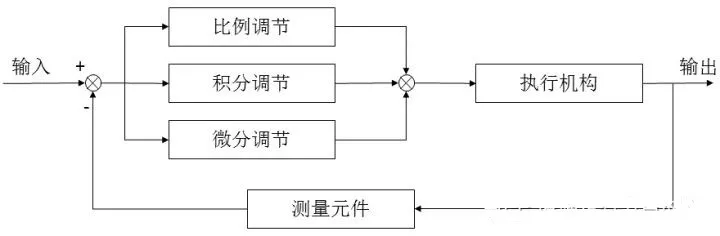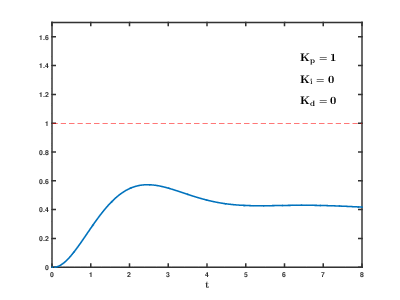# 抱歉，您的浏览器无法访问本站

Kelecn

“唯爱与科技不可辜负!”

### 一、什么是PID算法

PID，就是“比例（proportional）、积分（integral）、微分（derivative）”，是一种很常见的控制算法。常用于需要将某一个物理量“保持稳定”的场合（比如维持平衡，稳定温度、转速等）。### 二、PID算法原理

$$U(t)=K_P[e(t)+\frac{1}{T_I}\int{e(t)}dt+\frac{T_De(t)}{dt}]$$

Kp：控制器的比例系数。
Ti：控制器的积分时间，也称积分系数。
Td：控制器的微分时间，也称微分系数。1、P - 比例部分

2、I - 积分部分

3、D - 微分部分### 三、PID算法应用实例

PID 控制算法可以分为位置式 PID增量式 PID 控制算法。

（1）位置式PID控制的输出与整个过去的状态有关，用到了误差的累加值;而增量式PID的输出只与当前拍和前两拍的误差有关，因此位置式PID控制的累积误差相对更大;

（2）增量式PID控制输出的是控制量增量，并无积分作用，因此该方法适用于执行机构带积分部件的对象，如步进电机等，而位置式PID适用于执行机构不带积分部件的对象，如电液伺服阀。

（3）由于增量式PID输出的是控制量增量，如果计算机出现故障，误动作影响较小，而执行机构本身有记忆功能，可仍保持原位，不会严重影响系统的工作，而位置式的输出直接对应对象的输出，因此对系统影响较大。

1.位置式PID

$$u(k)=K_Pe(k)+K_I\sum_{i=0}{e(i)}+K_D[e(k)-e(k-1)]$$

2.增量式PID

$$\Delta u(k)=u(k)-u(k-1)=K_P[e(k)-e(k-1)]+K_Ie(k)+K_D[e(k)-2e(k-1)-e(k-2)]$$

PID原理和参数调试